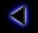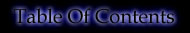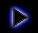.
How to calculate the Subnet Mask ?
.
This is the aim of the Mask Calculator. It helps you find out how big you need the Subnet part to be and how big you need the Host part to be, depending on your needs.
.
The Subnet Mask and Class determines how many subnets and hosts you get (i.e. how you subnet you network).
.
The number of hosts is: 2^(number of host bits)-2
All host bits to "0" is the "Subnet Address" (also called "Network address" or "Network") and all to "1" is the Broadcast Address.
.
The number of subnets is: 2^(number of subnet bits) if we tolerate all "0"s and all "1"s
or: 2^(number of subnet bits)-2 if not
.
For example:
A Class B IP subneted has follows: nnnnnnnn.nnnnnnnn.sssssshh.hhhhhhhh
(where "n"s represent the Network, "s"s the Subnet and "h"s the Host)
Has got: 2^6=64 subnets and 2^10-2=1022 hosts
For each subnet: